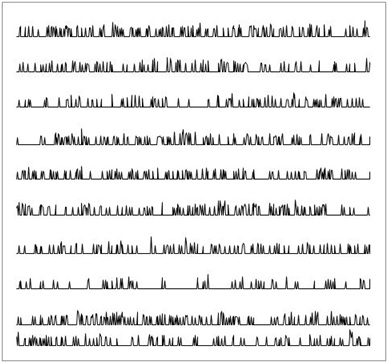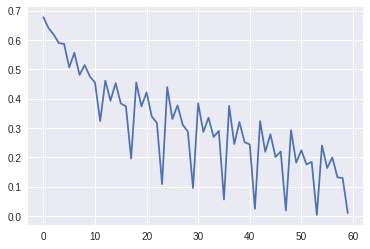# Implementing LSTM-FCN in pytorch - Part II

The follwoing article continues on the training of Multivariate LSTM-FCN architecture in pytorch. Part I details the implementatin of this architecture.

## Data

The dataset used for training the LSTM-FCN timeseries classifier is the Earthquake Dataset. In this classification problem we aim to predict whether a major event is about to happen based on a history of recent hourly readings taken between Dec 1st 1967, and 2003. The original sensor reading were transformed into a classification problem by:

• Definining a major event (what need to be predicted) as any reading above value 5 Rictor scale and then making sure this event is not aftershock of another major event.
• Constructing negative cases where there is a reading below 4 which was preceded by 20 or more non -zero readings in the last 512 hours.
• Segmenting the readings (rather than using a sliding window) so that they do not overlap in time.$curl -O http://www.timeseriesclassification.com/Downloads/Earthquakes.zip$ unzip Earthquakes.zip


Create pytorch DataLoaders for the training sets (should be the same for test set).

## Training

Before training the previously defined pytorch model, we need to implement a learner that will use an optimization algorithm (here Adam) to update the weights (actually the gradients that will be extracted from the weights) in a way that decreases the loss as follows:

As the output of the model is a hot encoded array (with number of classes as second dimension), and depending on the number of classes, we use a Binary Cross Entropy (a simple if/else statement) which is defined as follows:

$−(y * \log (\hat{y}) + (1−y) * \log (1−\hat{y}))$

For more than two classes or a Negative Log Likelihood loss which is known as Categorical Cross Entropy and is defined in a general manner by the function:

$− \sum_c y_c \log (\hat{y}_c)$

Then create the learner and train the model

The plot of the losses over batches should look like thisFull notebook can be found here - link